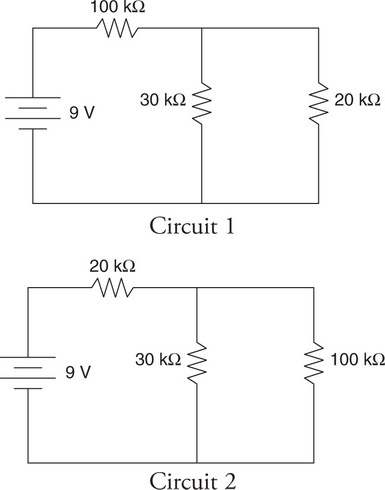# AP Physics 1 Question 171: Answer and Explanation

### Test Information

Question: 171

1.The circuits shown in the figure contain the same three resistors connected in different configurations. Which of the following correctly explains which configuration (if either) produces the larger current coming from the battery?

• A. Circuit 1; the larger resistor is closer to the battery.
• B. Circuit 2; the equivalent resistance of the three resistors is smaller than in circuit 1.
• C. Neither; the batteries are both the same.
• D. Neither; the individual resistors are the same in each circuit, even if they are in a different order.